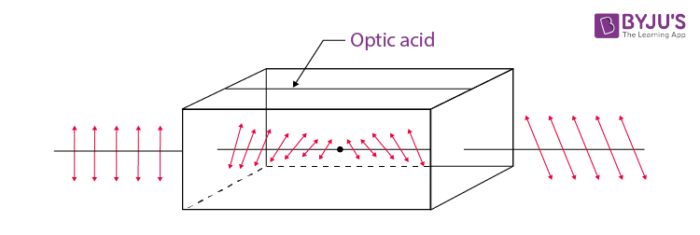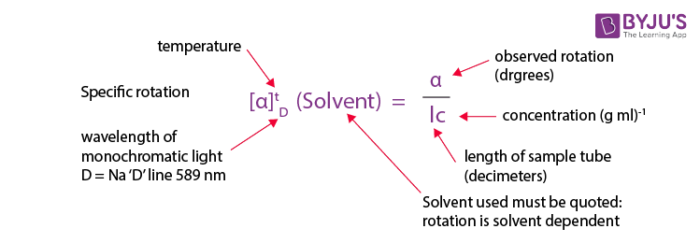# Optical Rotation (Optical Activity)

## What is Optical Rotation?

The optical rotation is the angle through which the plane of polarization is rotated when polarized light passes through a layer of liquid.

## What is Optical Activity?

Optical activity is the ability of a compound to rotate the plane of polarized light.

Organic materials typically exhibit optical activity. For instance, the sugar solution exhibits optical rotation when observed through a polarimeter because it is optically active. This property arises from an interaction of the electromagnetic radiation of polarized light with the unsymmetric electric fields generated by the electrons in a chiral molecule.

A compound is said to be optically active when the linearly polarized light is being rotated when it is passing through it. The optical rotation is the angle through which the plane of polarization is rotated when polarized light passes through a layer of a liquid. Optical rotation is the effect which is determined by the concentration of chiral molecules and their molecular structure in a substance. Every optical active substance has its own specific rotation.

## Optical Rotation Theory

The optical rotation that is the rotation of the plane of polarized light is shown below.The ability to rotate the plane of polarization of plane-polarized light by a certain substance is called optical activity. Substances that have the ability to rotate the plane of the polarized light passing through them are called optically active substances. Quartz and cinnabar are examples of optically active crystals while aqueous solutions of sugar, tartaric acid are optically active solutions.

Optically active substances are classified into two types.

1. Dextrorotatory substances – Substances that rotate the plane of polarization of the light towards the right are known as right-handed or dextrorotatory.
2. Laevorotatory substances – Substances which rotate the plane of polarization of the light toward the left are known as left-handed or

## Specific Optical Rotation

The specific optical rotation of a liquid substance is the angle of rotation measured as specified in the monograph, calculated with reference to a layer 100mm thick and divided by the relative density measured at the temperature at which the rotation is measured.

A measure of the optical activity of a sample is the rotation produced for a 1mm slab for a solid or a 100mm path length for a liquid. This measure is called the specific rotation. Liquids usually rotate the light much less than solids. Solutions of solids will obviously show an effect that depends on the concentration of active material and to a small extent, both on temperature and the solvent.

## The Formula of Optical Rotation

Optical activity is the ability of a compound to rotate the plane of polarized light. This property arises from an interaction of the electromagnetic radiation of polarized light with the unsymmetric electric fields generated by the electrons in a chiral molecule.

The rotation observed will clearly depend on the number of molecules exerting their effect that depends upon the concentrations. Observed rotations are thus converted into specific rotations that are a characteristic of the compounds according to the formula below.## Applications of Optical Rotation

• Optical rotation is a function of time and it is used to determine kinetic reactions
• This is also used to plot optical rotatory dispersion curves for a various range of wavelengths this helps in analysing molecular structure
• The optical rotation is measured on a layer of suitable thickness at the wavelength specified in the monograph.
• If the specific rotation of a sample is known its concentration in the solution can be estimated.
• If the concentration of the material in the sample is known then its specific rotation can be determined.
• The technique may be extended to the determination of optical substances in the presence of optically inactive species.

## Frequently Asked Questions – FAQs

Q1

### How do you measure optical rotation?

In an instrument called a polarimeter, optical rotation is measured. A linear association occurs between the rotation observed and the optically active compound concentration in the sample. The observed rotation and the wavelength of light used have a nonlinear interaction.

Q2

### How does concentration affect optical rotation?

The more the sample is condensed (the more molecules per volume unit), the more molecules are encountered. It would have greater optical rotations of condensed solutions and neat tests than dilute solutions.

Q3

### What is the angle of rotation in the polarimeter?

A polarimeter is a scientific instrument used to determine the angle of rotation caused by an optically active material moving through polarized light. As the angle of rotation is defined, the degree by which the light is rotated. Basically, the angle of rotation is known as the angle observed.

Q4

### What is the use of optical rotation?

It can also be used to test the purity of the material (absence of foreign compounds that are optically non-active) and as an assay technique. As polarized light travels through a film of a liquid, the optical rotation is the angle through which the polarization plane is rotated.

Q5

### How does temperature affect optical rotation?

That being said, temperature will influence how the material interacts with light, and there would be no significant difference in precise rotation in most typical solutions at mild temperatures. This optical behaviour affects the temperature. This depends on the light wavelength used and on the material.

Take up a quiz on Optical rotation